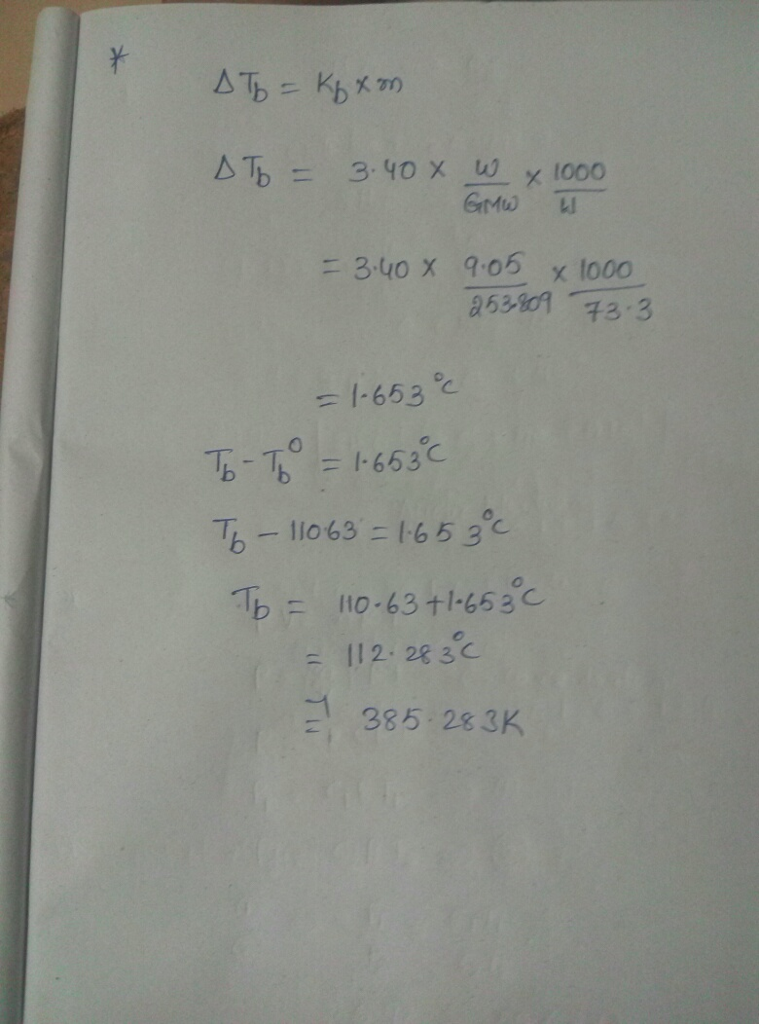# Question & Answer: What is the boiling point (in °C) of a solution of 9.05 g of I2 in 73.3 g of toluene, assuming the…..

What is the boiling point (in °C) of a solution of 9.05 g of I2 in 73.3 g of toluene, assuming the I2 is nonvolatile? (For toluene, Tb = 110.63°C and Kb = 3.40°C·kg/mol.)